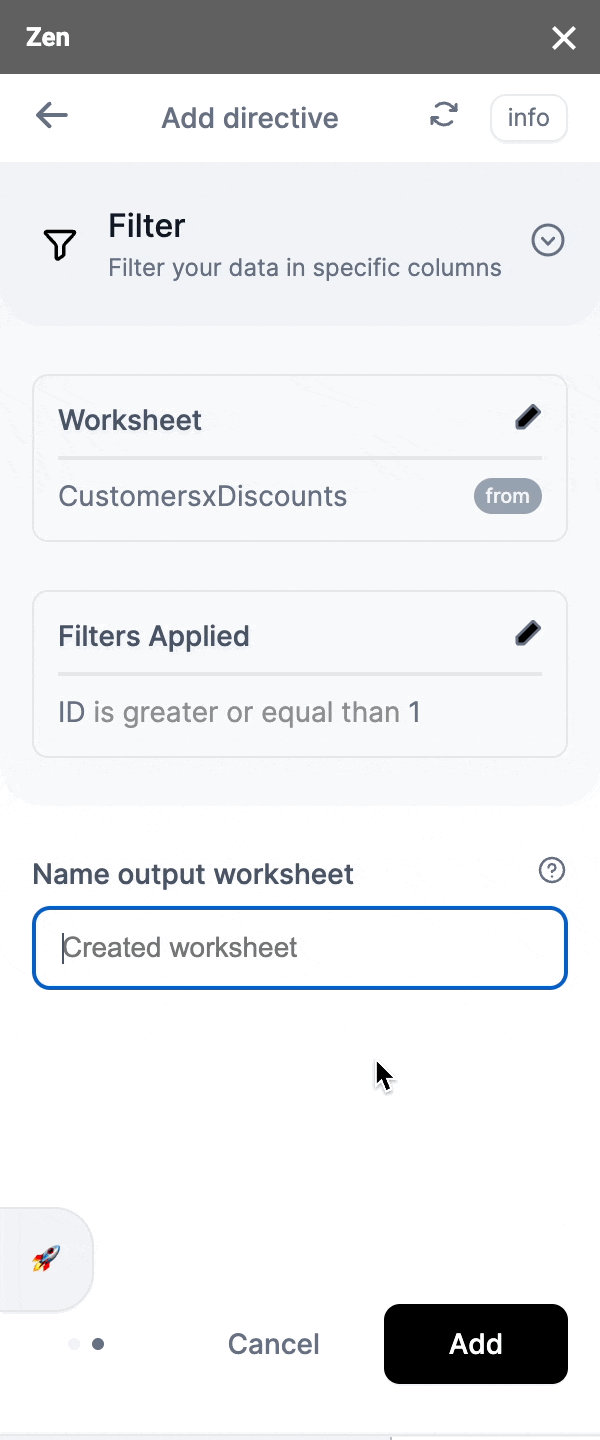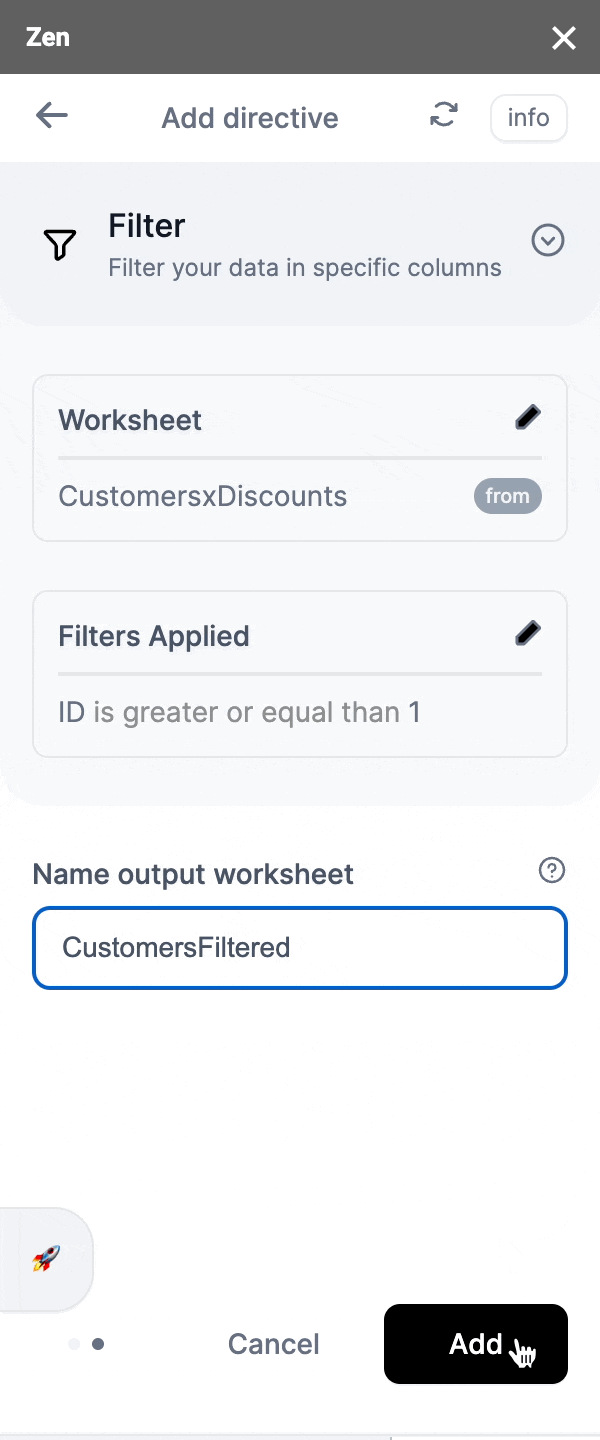# filter

Filter your data and selectively choose rows from a worksheet. A step-by-step.

The filter directive is powerful. It allows the user to selectively choose data rows from a worksheet. Being able to select rows from a worksheet can simplify data processing.

For instance, a user could filter out high-value customers from a list of customers, or in another scenario, filter out "buy" transactions from a list of stock transactions.

📘

Important Note

Columns can be filtered by value (e.g. 1, 30, 1029, etc), text strings (e.g. A, New, AB) and by targeting another column (e.g. Column1 is equal to Column2)

## Types of filters

👍

What type of data can you filter?

You can filter different types of data with Zen, here are some of them:

• numbers in numeric order
• text in alphabetical or alphanumeric order (words, names, emails, addresses, etc)
• dates in temporal order
• cell comparison (compare to a value in a cell whether number, text, or date)
Numbers ExampleText strings Example
is equal to

(Symbol: =)
"ColumnName is equal to 2"

Results will only contain data in ColumnName that is 2.
"ColumnName is equal to A"

Results will only contain data in ColumnName that is A.
is not equal to

(Symbol: ≠)
"ColumnName is not equal to 1"

Results will contain all data in ColumnName other than 1.
"ColumnName is not equal to B"

Results will contain all data in ColumnName other than B.
is greater than

(Symbol: >)
"ColumnName is greater than 1"

Results will contain all data in ColumnnName that is 2 and above.
"ColumnName is greater than B"

Results will contain all data in ColumnName that is after B (according to the alphabets)
is greater than or equal to

(Symbol: ≥)
"ColumnName is greater than or equal to 1"

Results will contain all data in ColumnName that is 1 and above.
"ColumnName is greater than or equal to B"

Results will contain all data in ColumnName that is B and after (according to the alphabets).
is less than

(Symbol: <)
"ColumnName is less than 10"

Results will contain all data in ColumnName that is 9 and below.
"ColumnName is less than D"

Results will contain all data in ColumnName that is before D (according to the alphabets).
is less than or equal to

(Symbol: ≤)
"ColumnName is less than or equal to 10"

Results will contain all data in ColumnName that is 10 and below.
"ColumnName is less than or equal to D"

Results will contain all data in ColumnName that is D and before (according to the alphabets).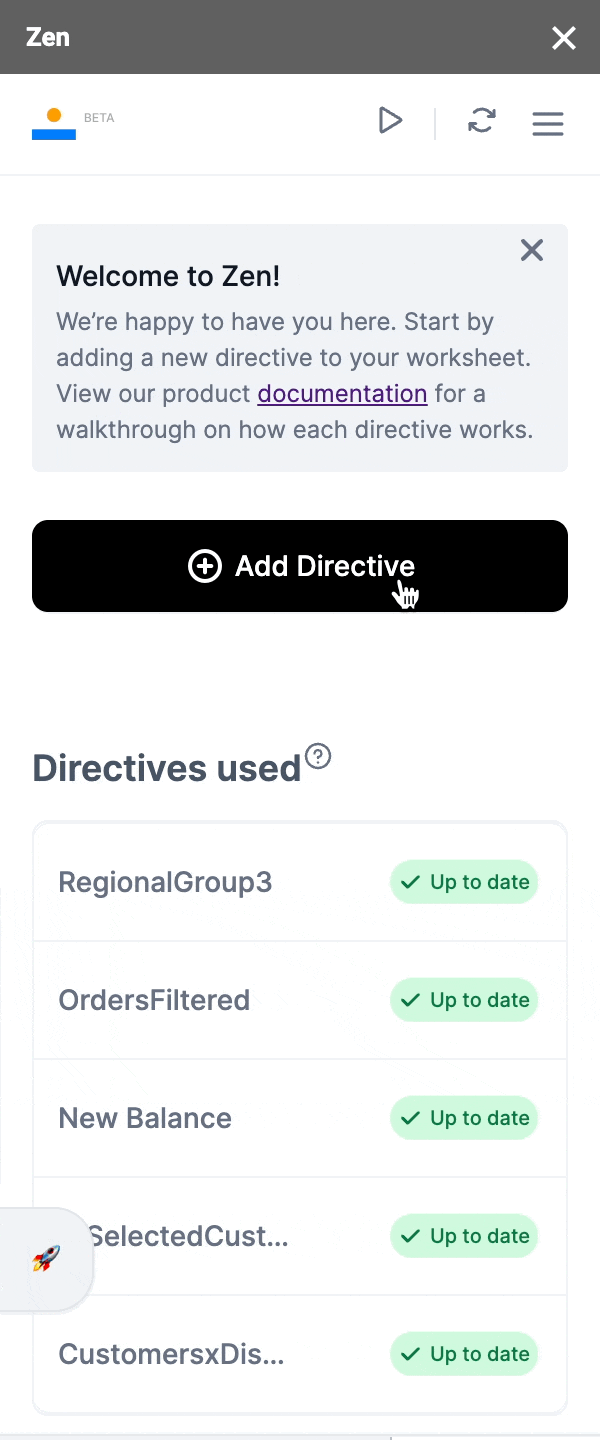Step 2: Choose "Filter"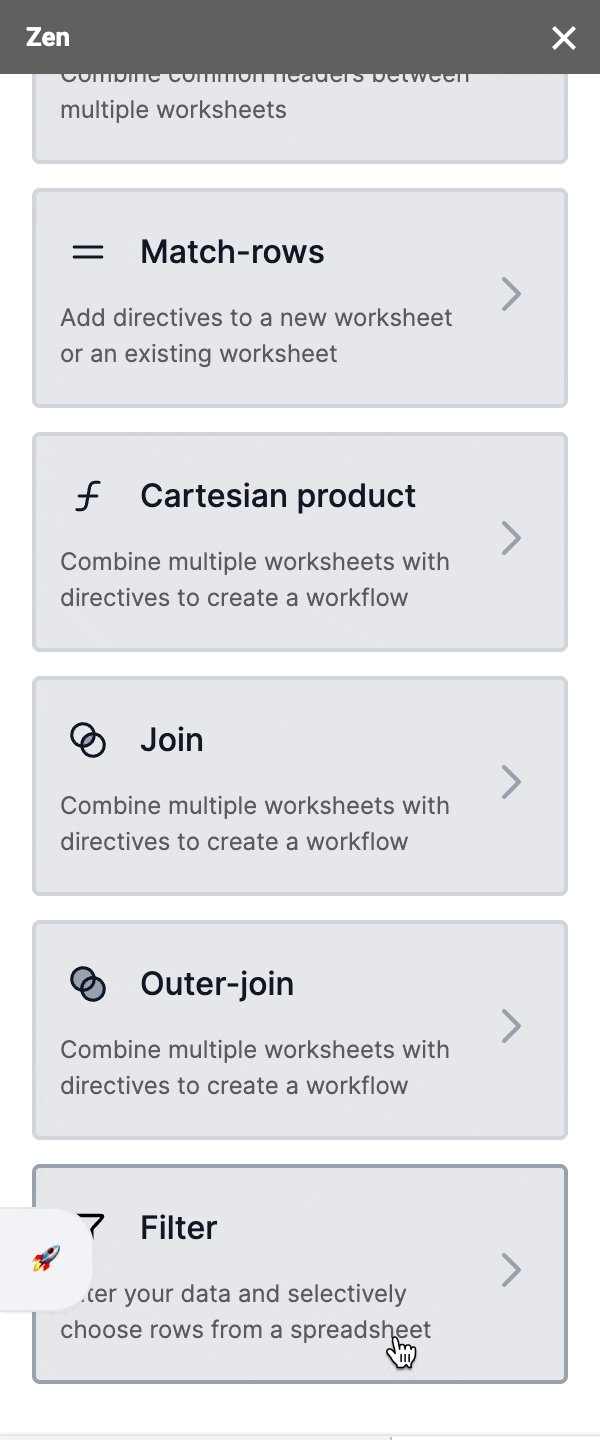Step 3: Select the worksheet you'd want to filter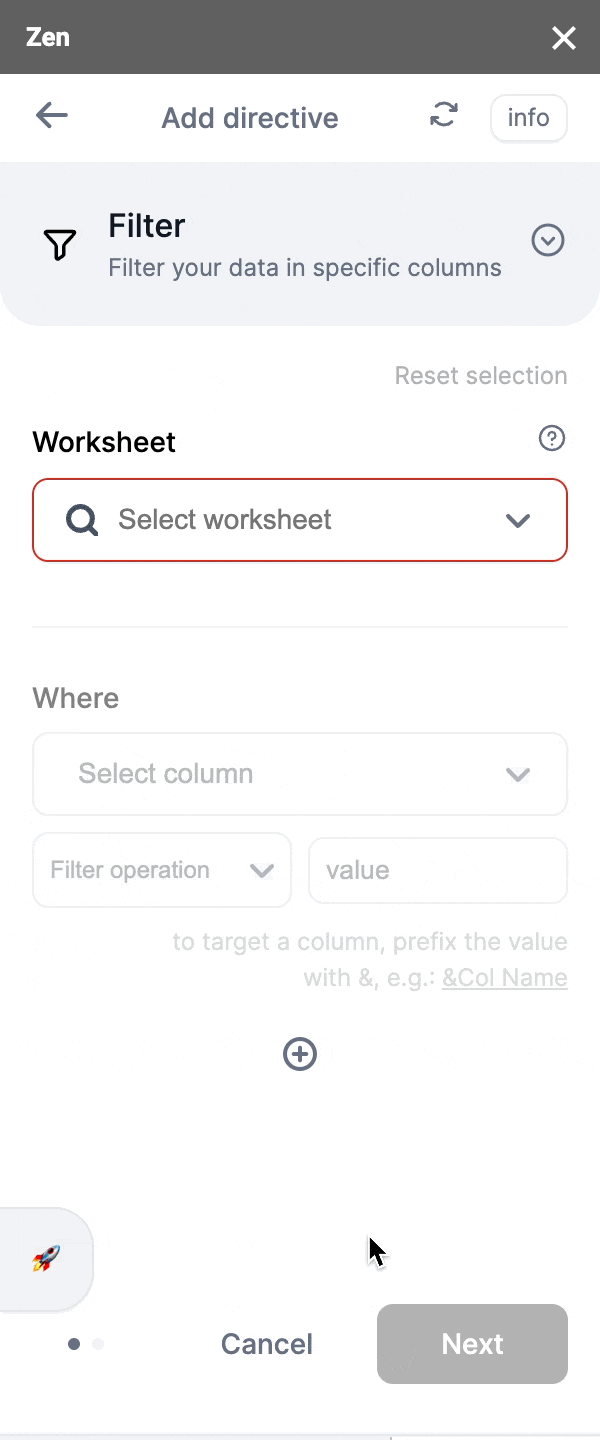Step 4: Select the column(s) you'd like to filter and how you'd like it filtered

👍

Filtering options

• Filter more than one column at a time
• Create filters for column comparison (e.g Column1 is equal to Column2)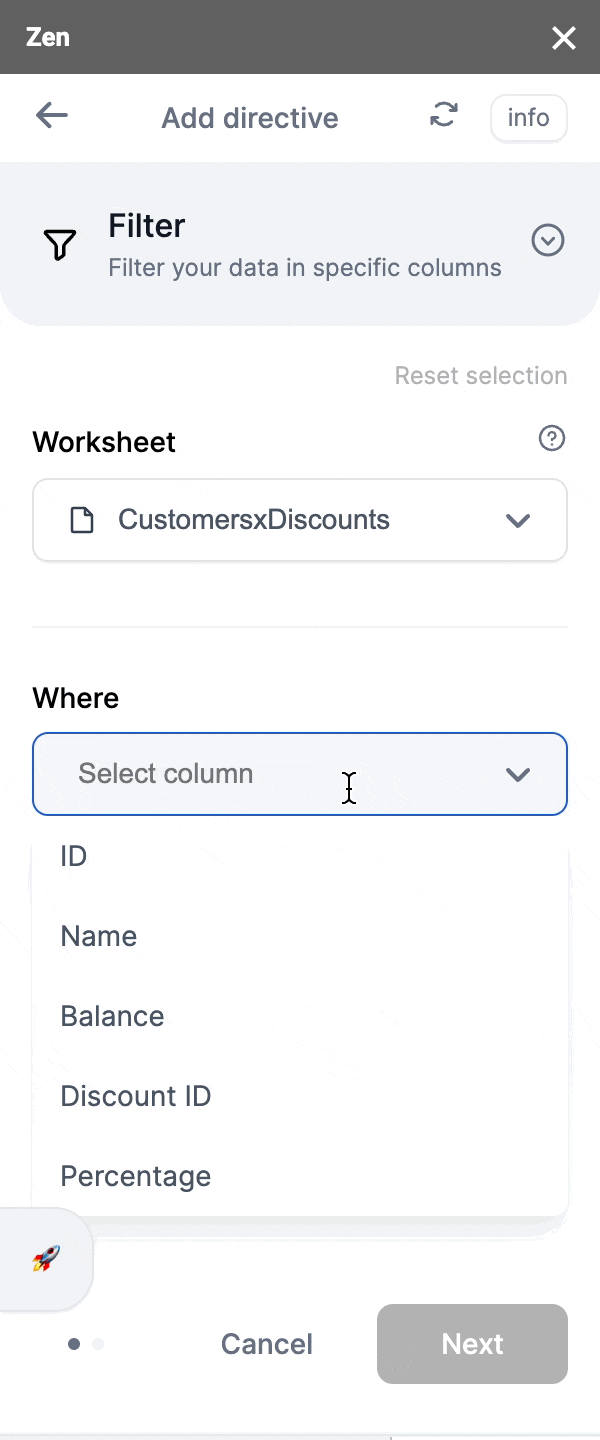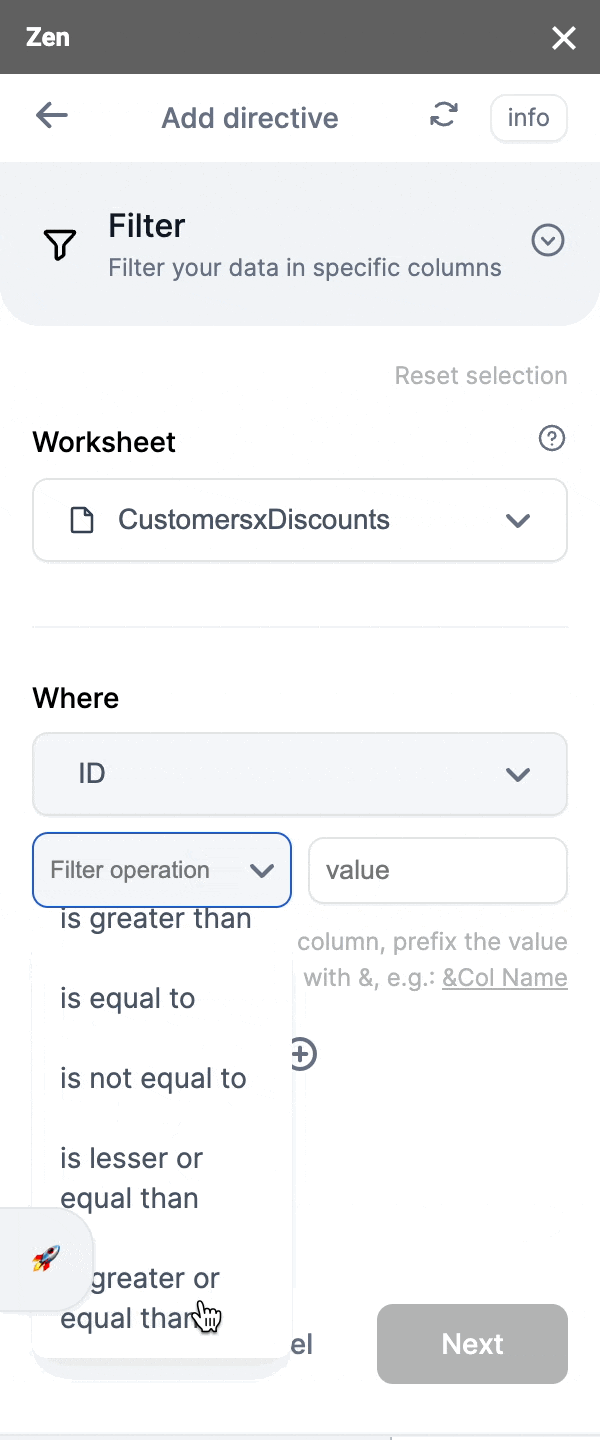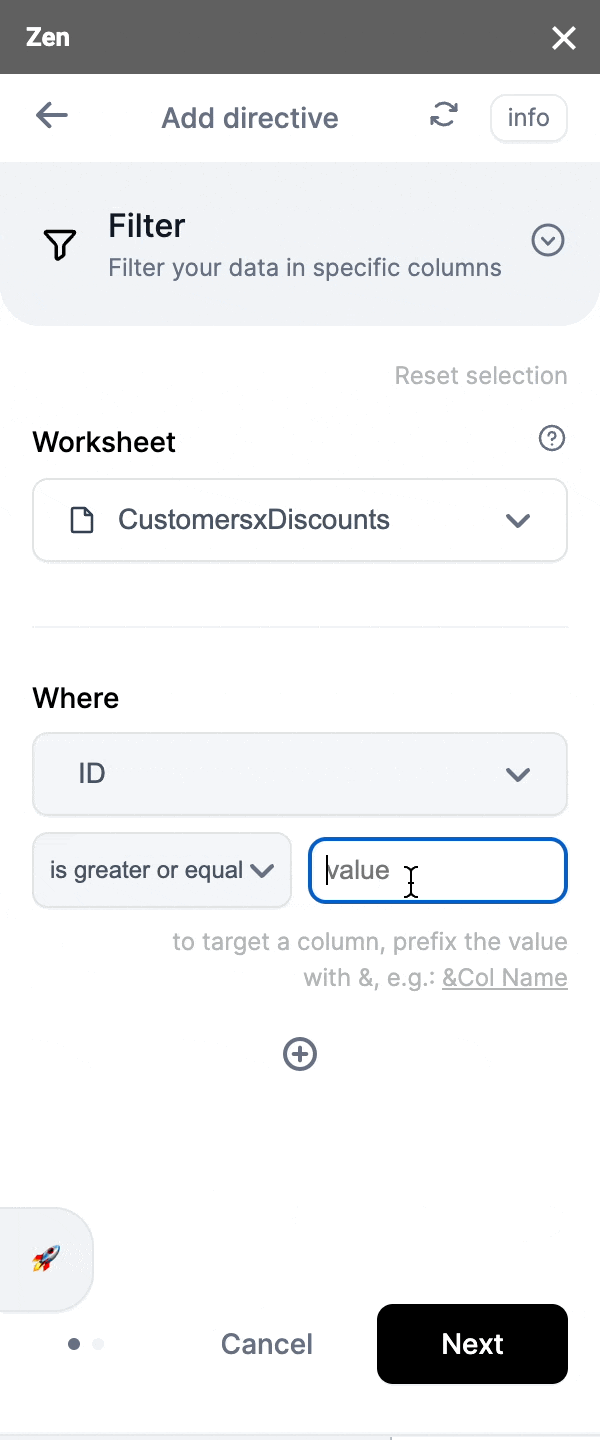Step 5: Once you're happy with your selection, click 'Next' and proceed to the next screen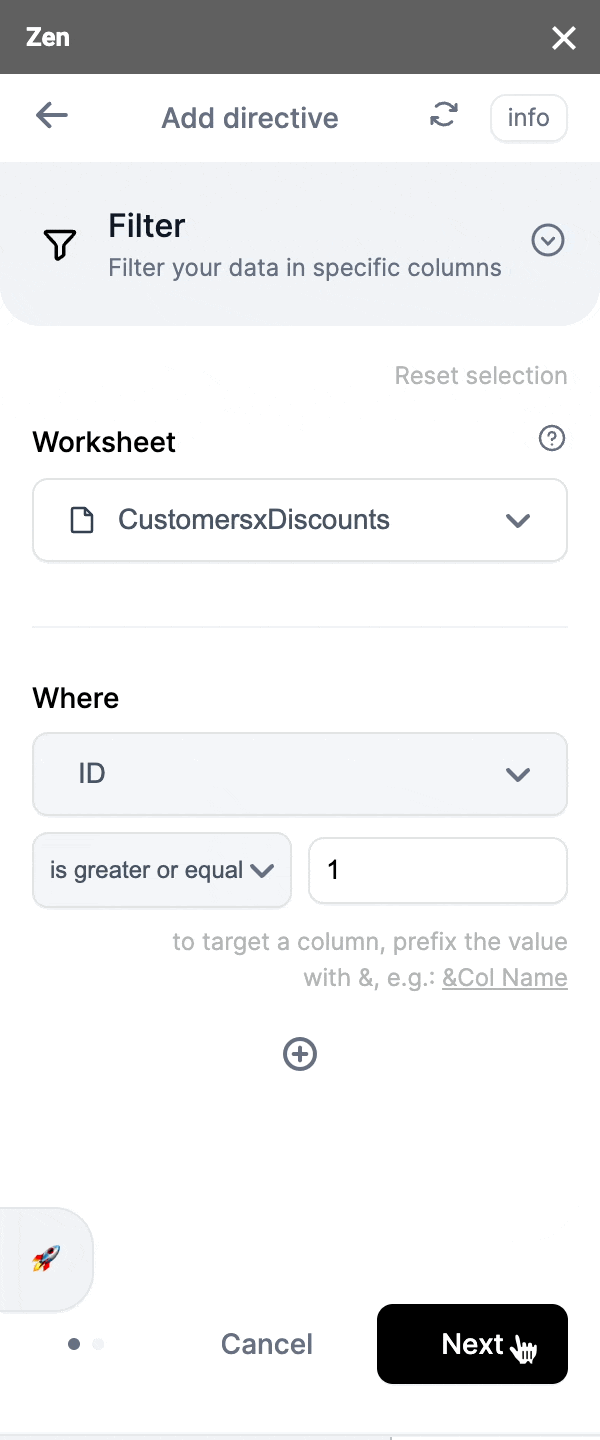Step 6: Name your output worksheet

This will be where your output data from connecting the worksheet by adding 'filter' will be.